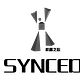# A Guide to Receptive Field Arithmetic for Convolutional Neural Networks

Blog Author: Đặng Hà Thế Hiển
Blog: https://medium.com/@nikasa1889/a-guide-to-receptive-field-arithmetic-for-convolutional-neural-networks-e0f514068807

# 1.Receptive Field and Feature Map Visualization

The receptive field is defined as the region in the input space that a particular CNN’s feature is looking at (i.e. be affected by).

For convolutional neural network, the number of output features in each dimension can be calculated by the following formula:

In this blog, the number of (input/output) features equals the amount of the features along one axis (one dimension) of the input/output. Here, an axis can be understood as the width, height or a channel of a color image.

Take the following figure as an example:

For the upper left sub-figure, the input image is a 5 x 5 matrix (blue grid). Then zero-padding with size of p = 1 (transparent grid around the input image) is used to maintain the edge information during convolution. After that, a 3 x 3 kernel with stride of s = 2 is used to convolve this image to gain itsfeature map (green grid) with size of 3 x 3. In this example, nine features are obtained and each feature has a receptive field with size of 3 x 3 (the area inside light blue lines). We can use the same convolution on this green grid to gain a deeper feature map (orange grid) as shown in sub-figure at the left bottom. As for orange feature map, each feature has a 7 x 7 receptive field.

The method above is a common way to visualize a CNN feature map. But if we only look at the feature map (green or orange grid), we cannot directly know which pixels a feature is looking at and how big is that region. The two sub-figures in the right column present another way to visualize the feature map, where the size of each feature map is fixed and equals to the size of input, and each feature is located at the center of its receptive field. In this situation, the only task is to calculate the area of the receptive field mathematically.

# 2. Receptive Field Arithmetic

Receptive field in each layer can be calculated by using the following equations:

The first equation is defined by the same way as the first part of this article.

The second equation calculates the jump (j) in the output feature map. The jump is the distance between two adjacent features. For an original input image, jump equals 1.

The third equation calculates the size of receptive field (r) of one output feature.

The fourth equation calculates the center position of the receptive field of the first output feature. Here, start is the center coordinate of one pixel.

The following figure shows the details of receptive field computation procedure:

# 3. Code for Receptive Field Computation

The blog author also creates a small python program that can calculate the receptive field information (full code: https://gist.github.com/Nikasa1889/781a8eb20c5b32f8e378353cde4daa51#file-computereceptivefield-py).

`def outFromIn(conv, layerIn):      n_in = layerIn      j_in = layerIn      r_in = layerIn      start_in = layerIn      k = conv      s = conv      p = conv            n_out = math.floor((n_in - k + 2*p)/s) + 1      actualP = (n_out-1)*s - n_in + k       pR = math.ceil(actualP/2)      pL = math.floor(actualP/2)            j_out = j_in * s      r_out = r_in + (k - 1)*j_in      start_out = start_in + ((k-1)/2 - pL)*j_in      return n_out, j_out, r_out, start_out`

The following figure shows the output of this program on AlexNet:

This blog is inspired by the paper A guide to convolution arithmetic for deep learning(https://arxiv.org/pdf/1603.07285.pdf). This guide provides an intuitive understanding of the relationship between input, kernel, zero-padding, strides and output in convolutional, pooling and transposed convolutional layers. Following GIFs shows some basic computation in convolutional neural networks:

Convolution without zero-padding and with stride of 1:

Convolution with zero-padding and with stride of 1:

Convolution with zero-padding and with stride of 2 (used in this blog):

(Gif source: https://github.com/vdumoulin/conv_arithmetic)

This blog is a good guide on how to calculate and visualize the receptive field information of a convolutional neural network. The intuitive examples in this guide can help beginners understand the architecture of a deep network better.

There is also some further works for visualization of hidden layers, for example the paper Visualizing and Comparing AlexNet and VGG using Deconvolutional Layers(https://icmlviz.github.io/assets/papers/4.pdf).

All these works aim at visualizing what convolutional neural networks learn. Besides the visualization of receptive fields, the visualization of Activation-Layers or visualization of filters/weights is also good ways to understand hidden layers in deep CNNs as shown in following figures (source: http://cs231n.github.io/understanding-cnn/):

Author: Yiwen Liao| Editor: Junpei Zhong|Localized by Synced Global Team: Xiang Chen

Written by

Written by

## Synced

#### AI Technology & Industry Review — syncedreview.com | Newsletter: http://bit.ly/2IYL6Y2 | Share My Research http://bit.ly/2TrUPMI | Twitter: @Synced_Global